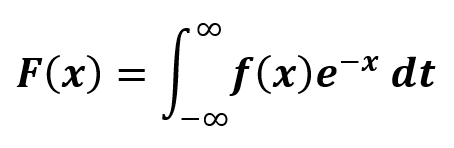# Python | Fast Fourier Transformation

• Difficulty Level : Medium
• Last Updated : 26 Aug, 2019

It is an algorithm which plays a very important role in the computation of the Discrete Fourier Transform of a sequence. It converts a space or time signal to signal of the frequency domain.
The DFT signal is generated by the distribution of value sequences to different frequency component. Working directly to convert on Fourier transform is computationally too expensive. So, Fast Fourier transform is used as it rapidly computes by factorizing the DFT matrix as the product of sparse factors. As a result, it reduces the DFT computation complexity from O(n2) to O(N log N). And this is a huge difference when working on a large dataset. Also, FFT algorithms are very accurate as compared to the DFT definition directly, in the presence of round-off error.

This transformation is a translation from the configuration space to frequency space and this is very important in terms of exploring both transformations of certain problems for more efficient computation and in exploring the power spectrum of a signal. This translation can be from xn to Xk. It is converting spatial or temporal data into the frequency domain data.## sympy.discrete.transforms.fft( ) :

It can perform Discrete Fourier Transform (DFT) in the complex domain.
Automatically the sequence is padded with zero to the right because the radix-2 FFT requires the sample point number as a power of 2. For short sequences use this method with default arguments only as with the size of the sequence, the complexity of expressions increases.

```
Parameters :
-> seq : [iterable] sequence on which DFT is to be applied.
-> dps : [Integer] number of decimal digits for precision.

Returns :
Fast Fourier Transform
```

Example 1 :

 `# import sympy ``from` `sympy ``import` `fft`` ` `# sequence ``seq ``=` `[``15``, ``21``, ``13``, ``44``]`` ` `# fft``transform ``=` `fft(seq)``print` `(transform)`

Output :

`FFT : [93, 2 - 23*I, -37, 2 + 23*I]`

Example 2 :

 `# import sympy ``from` `sympy ``import` `fft`` ` `# sequence ``seq ``=` `[``15``, ``21``, ``13``, ``44``]`` ` `decimal_point ``=` `4`` ` `# fft``transform ``=` `fft(seq, decimal_point )``print` `(``"FFT : "``, transform)`

Output :

```FFT :  [93, 2.0 - 23.0*I, -37, 2.0 + 23.0*I]
```

My Personal Notes arrow_drop_up